## Getting Started with Creating Charts in Python

Started ‎04-12-2016 by
Modified ‎04-16-2019 by
Views 845

# Getting Started with Creating Charts in Python

There are many Python packages available for creating charts. Which one you use really depends on what the purpose of the final plot is. For quick results, Pandas and Seaborn are quite popular. For publication-ready plots, Matplotlib is a very common choice (the previous two packages are actually wrappers around Matplotlib). And for interactive plots, you may want to try Plot.ly or Bokeh.

The first thing we need to do is connect to CAS and upload some data. We are using the SAS CARS dataset in CSV form here.

``````In: import swat

In: conn = swat.CAS()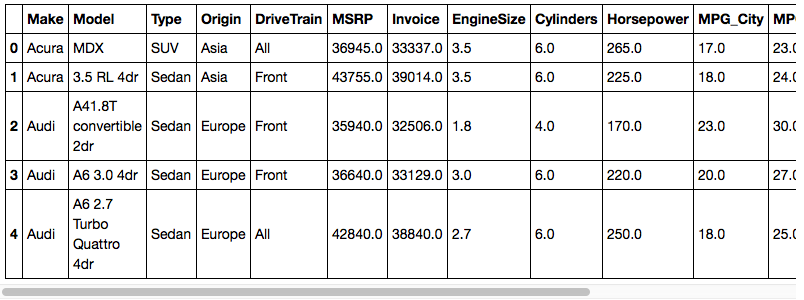Let's subset the data to just the sports cars using the query method of the CASTable object.  This works just like the query method on DataFrames.  We'll then download the data into a local DataFrame using the head method.  We've specified a maximum numer of rows as 1,000 here.  That will cover all of the sports cars in the result.  Finally, we'll add an index to the DataFrame that contains the make and model of the car.

``````In: sports = tbl.query('Type = "Sports"')
In: sports
Out: CASTable('_PY_c46200ed_1bad_4135_8b14_031a3890445c_', caslib='CASUSERHDFS(kesmit)', where='Type = "Sports"')

In: df.set_index(df['Make'] + ' ' + df['Model'], inplace=True)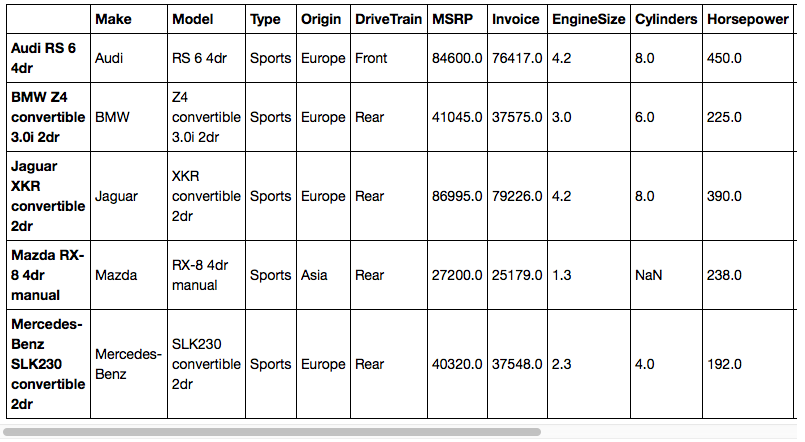Now that we have some data to work with, let's create some charts. To enable Matplotlib to embed images directly in the notebook, use the `%matplotlib `magic command. This works with Pandas plotting, Seaborn, and Matplotlib charts.

``In: %matplotlib inline``

## Pandas plot Method

Pandas DataFrames have a property called plot that makes it easy to create quick charts from the data in the DataFrame. In older versions of Pandas, plot was a method with a kind= attribute that indicated the type of plot to create. Newer versions of plot have methods for each individual plot type such as bar, scatter, line, etc.

In the example below, we are subsetting the DataFrame to only include MSRP and Invoice, then we are calling the plot.bar method to create bar charts of the columns in subplots. We will also use the rot= parameter to rotate the x axis labels.

``In: df[['MSRP', 'Invoice']].plot.bar(figsize=(15, 8), rot=-90, subplots=True)``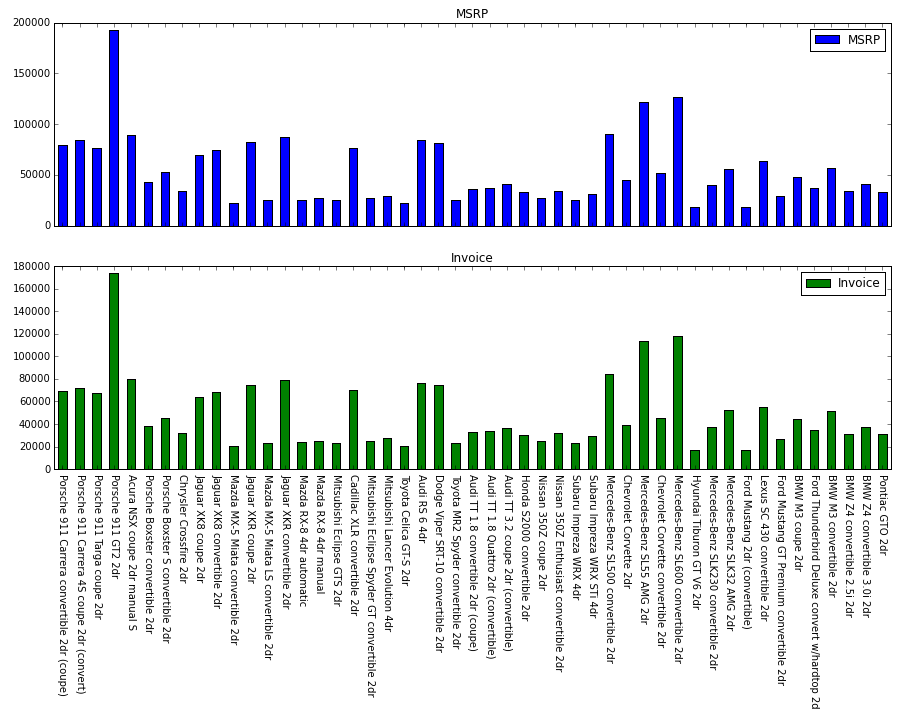## Creating Charts using Seaborn

The next step up from the plot method of DataFrames is using the Seaborn package. This package is a wrapper around Matplotlib that takes some of the work out of creating graphs and adds new ways of styling charts.

The code below creates a figure that contains two subplots as we did before. Seaborn is then used to create bar charts in each of the axes. Finally, the x axis labels are overridden so that they can be rotated -90 degrees as we did before.

``````In: import seaborn as sns
In: import matplotlib.pyplot as plt

In: f, (ax1, ax2) = plt.subplots(2, 1, figsize=(15, 8), sharex=True)

In: bar = sns.barplot(df.index, df['MSRP'], ax=ax1, color='blue')
In: ax1.set_ylabel('MSRP')

In: bar2 = sns.barplot(df.index, df['Invoice'], ax=ax2, color='green')
In: ax2.set_ylabel('Invoice')

In: labels = bar2.set_xticklabels(df.index, rotation=-90)``````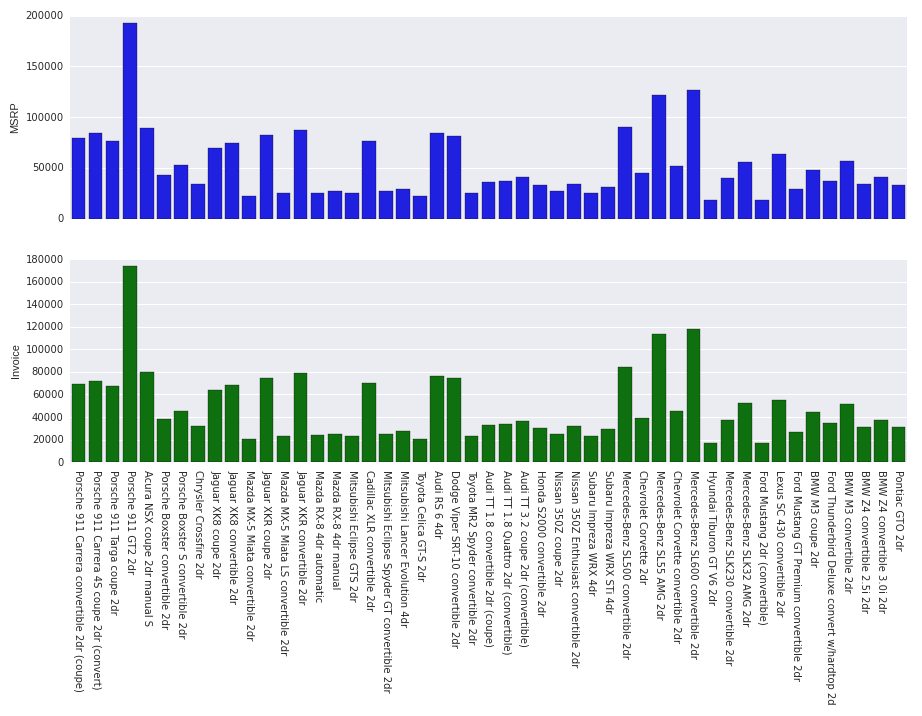## Using Matplotlib Directly

The final entry in the static graphics line is Matplotlib itself. Panda's plot method and Seaborn are just wrappers around Matplotlib, but you can still use Matplotlib directly. For this case, it doesn't look a lot different than the Seaborn case. You'll noticed that we have to do a bit more adjustment of labels on the x axis and the x axis is a bit wider than it needs to be. Seaborn just helps out with those details automatically.

``````In: import matplotlib.pyplot as plt

In: f, (ax1, ax2) = plt.subplots(2, 1, figsize=(15, 8), sharex=True)

In: ax1.bar(range(len(df.index)), df['MSRP'], color='blue')
In: ax1.set_ylabel('MSRP')

In: ax2.bar(range(len(df.index)), df['Invoice'], color='green')
In: ax2.set_ylabel('Invoice')

In: ax2.set_xticks([x + 0.25 for x in range(len(df.index))])
In: labels = ax2.set_xticklabels(df.index, rotation=-90)``````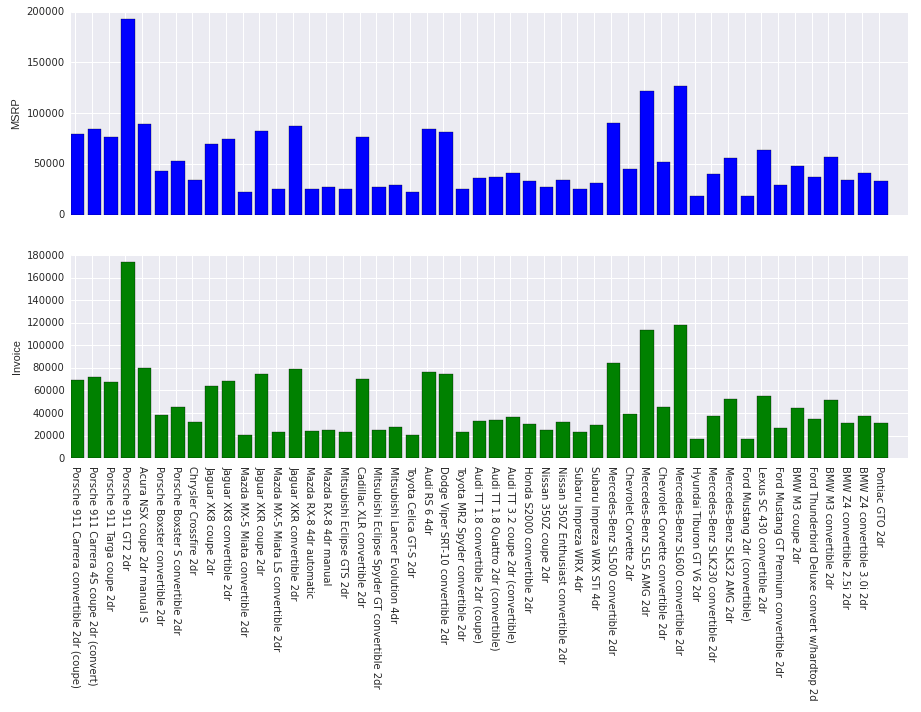The Plot.ly package can be used a couple of different ways. There's the Plot.ly API that uses standard Python structures as inputs, and there is an additional package called Cufflinks that integrates Plot.ly charts into Pandas DataFrames. Since we have our data in a DataFrame, it's easier to use Cufflinks to start.

The code below uses Cufflinks' iplot method on the DataFrame. The iplot method works much like the standard plot method on DataFrames except that it uses Plot.ly as the back-end rather than Matplotlib. After importing cufflinks, we use the go_offline function to indicate that we are using local graphics rather than the hosted Plot.ly service.

The benefit to Plot.ly graphics is that they are interactive when viewed in a web browser.

``````In: import cufflinks as cf

In: cf.go_offline()

In: df[['MSRP', 'Invoice']].iplot(kind='bar', subplots=True, shape=(2, 1), shared_xaxes=True)``````To do a similar plot using the standard Plot.ly API takes a bit more work.

``````In: import plotly.graph_objs as go
In: from plotly import tools
In: from plotly.offline import init_notebook_mode, iplot

In: init_notebook_mode()

In: data = [
go.Bar(x=df.index, y=df.MSRP, name='MSRP'),
go.Bar(x=df.index, y=df.Invoice, name='Invoice')
]

In: fig = tools.make_subplots(rows=2, cols=1, shared_xaxes=True, print_grid=True)
In: fig.append_trace(data, 1, 1)
In: fig.append_trace(data, 2, 1)

In: fig['layout']['height'] = 700
In: fig['layout']['margin'] = dict(b=250)

In: iplot(fig)``````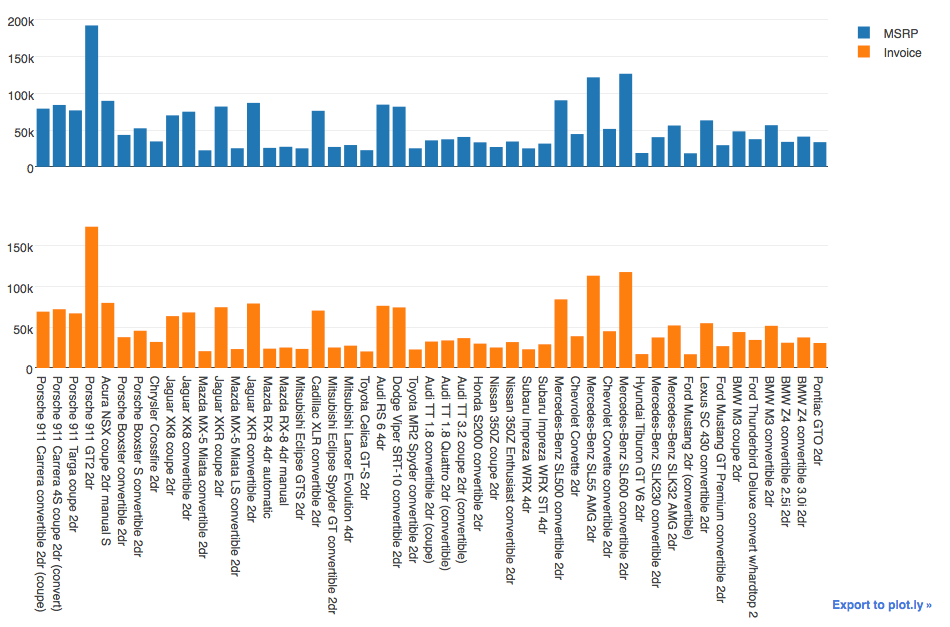## Creating Charts with Bokeh

Bokeh is a popular graphics library for Python. The charting functionality is a more recent addition, so it isn't as mature as some of the other libraries here. However, it is an extremeley powerful and popular Python package. This chart could still use some work with label orientation and doing the two pieces as subplots rather than separate plots, but the functionality doesn't appear to exist in this release.

``````In: from bokeh.charts import Bar, show
In: from bokeh.io import output_notebook

In: output_notebook()

In: show(Bar(df, values='MSRP', ylabel='MSRP', width=1000, height=400, color='blue'))
In: show(Bar(df, values='Invoice', ylabel='Invoice', width=1000, height=400, color='green'))``````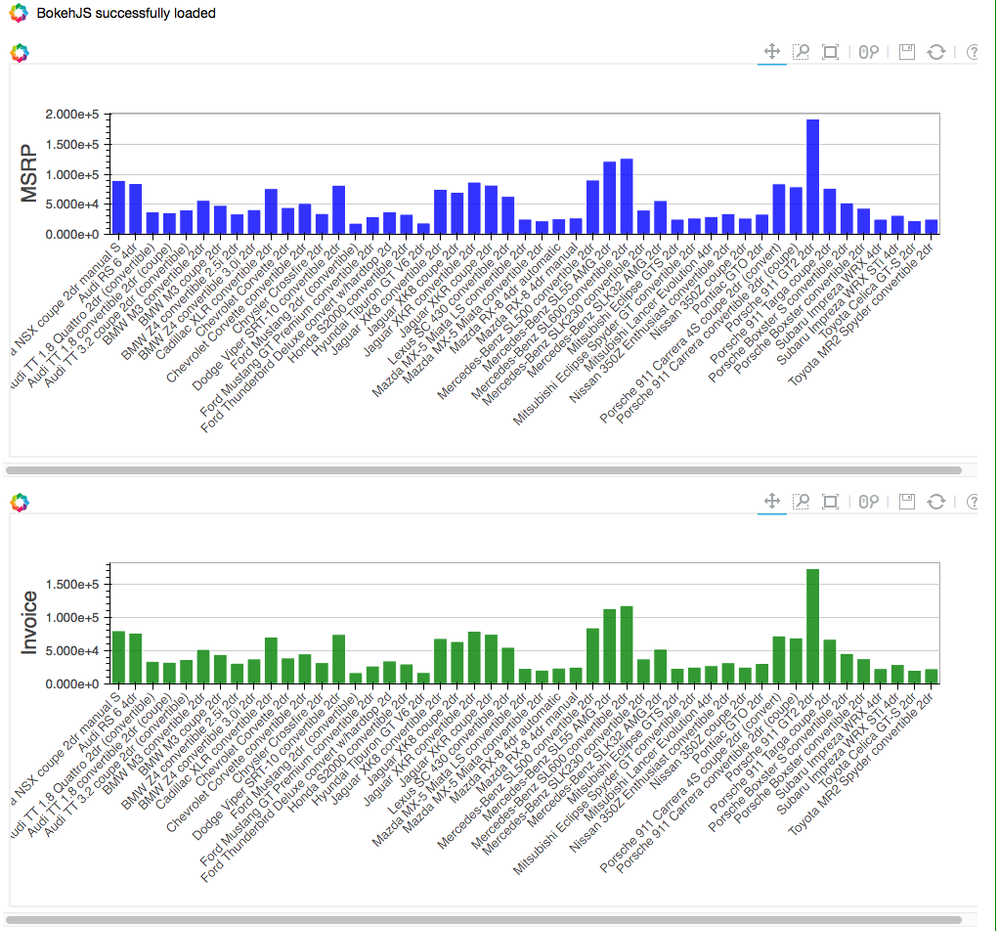Don't forget to close the connection when you're finished.

``In: conn.close()``

## Conclusion

We have shown the basics of several Python charting libraries here. Which of these (if any) that you use for your purposes really depends on your needs. The Matplotlib-based libraries are better at static and publication-style grahpics, whereas Plot.ly and Bokeh are more tuned to interactive charting in web browsers. Hopefully, we have given you enough information to pique your interest in one of these packages for creating charts from your CAS results.

## Resources